# Homework 10¶

## Homework Submission Workflow¶

When you submit your work, follow the instructions on the submission workflow page scrupulously for full credit.

Important: Failure to do any of the following will result in lost points:

• Submit one PDF file and one notebook per group

• Enter all the group members in your PDF Gradescope submission, not just in your documents. Look at this Piazza Post if you don't know how to do this

• Match each answer (not question!) with the appropriate page in Gradescope

• Avoid large blank spaces in your PDF file

### Allowed Python Libraries¶

Except for part 4, you may only import the numpy, matplotlib, and, if needed math modules for this assignment.

## Part 1: Correlation¶

### Problem 1.1¶

Let

$$\mathbf{x} = [1, 0, 2, -1, 3]^T \;\;\;\text{and}\;\;\; \mathbf{g} = [-1, 2, 1]^T\;.$$

Give the valid correlation $\mathbf{z}_v$ and the shape-preserving correlation $\mathbf{z}_s$ of signal $\mathbf{x}$ with kernel $\mathbf{g}$.

### Problem 1.2¶

Using the values in the previous problem, give the matrices $V_v$ and $V_s$ such that

$$\mathbf{z}_v = V_v \mathbf{x} \;\;\;\text{and}\;\;\; \mathbf{z}_s = V_s \mathbf{x} \;.$$

### Problem 1.3¶

Let

$$X = \left[\begin{array}{rrrrr} 2 & 1 & 3 & -1 & 4 \\ 0 & -2 & 1 & 0 & 3 \end{array}\right] \;\;\;\text{and}\;\;\; G = \left[\begin{array}{rr} 1 & 2 \\ -3 & 0 \end{array}\right]\;.$$

Give the valid correlation $Z_v$ and the shape-preserving correlation $Z_s$ of image $X$ with kernel $G$.

### Problem 1.4¶

Give the image $Z_v$ as defined in the previous problem, except that stride 2 is used.

## Part 2: Layers¶

The code below implements a generic Layer class for a neural network, and a fully connected layer class FCLayer. This is a rather stripped down and somewhat inefficient implementation, introduced here for pedagogical purposes. This implementation is described next. Please examine the code as you read the description. It is unlikely that this description will make sense to you unless you have read and understood the class notes on the architecture of neural nets and on back-propagation.

• The function tensorize is introduced as a way to cope with the poor way in which numpy handles matrix products, now that the matrix data type is being phased out. Tensorizing arguments passed to the various methods of the implementation ensures that they are floating-point numpy arrays with no singleton dimensions. Singleton dimensions are array shape components equal to 1. For instance, the second dimension of the array np.zeros((3, 1)) is a singleton dimension. The array is equal to array([[0.], [0.], [0.]]) and tensorize converts it to np.zeros(3), which is equal to array([0., 0., 0.]). With this change, the operation np.dot(a, b) is equivalent to matrix multiplication of a and b whenever a and b are tensorized and their matrix product is well defined (after tensorization). This is sufficient for working with Jacobians.

• The Layer class implements methods that all layers either use as is or override. These methods include __init__, which requires a minimum of methods for initialization, reset, which does nothing in its generic version, getWeights and setWeights, which respectively retrieve or set all the parameters in the layer, and dfdw, which computes the Jacobian of the output of the layer relative to its parameters.

• The method dfdw is provided as a default for layers that have no parameters. In that case, the method returns an empty array. Layers with parameters must override this method.

• The attribute parms of a layer is a list of parameter arrays. For instance, a FCLayer stores the gain matrix V and bias vector b in its parms attribute. Regardless of the number and shapes of the parameters in parms, the method getWeights always returns the parameters as a single vector, obtained by concatenating the flattened versions of all the parameters. For instance, if V is a $2\times 3$ array and b is a vector with two biases, then getWeights returns a single vector with 8 values.

• The attribute x is initially set to None. It is important to note that every layer remembers its input in this attribute. In this way, when the methods dfdw and dfdx are called to compute the Jacobian of a layer's output relative to either parameter vectors w or input x, the value of the input is known.

• The method setWeights takes a vector of parameters, assumed to have the correct number of elements, and reshapes it into a list of appropriately sized entries, which it binds to the parms attribute of the layer.

• The class FCLayer implements the affine part of a fully connected layer (that is, it does not include the nonlinearity, which is a separate layer). This class overrides __init__, dfdw, and reset, and provides an additional method ofShape.

• The __init__ method of FCLayer takes arguments V and b (a gain array and a bias vector) and initializes the layer. It also defines a method f, which takes a vector x and returns the output of the layer, and a method dfdx, which returns the Jacobian of the layer output relative to its input. If there are $e$ outputs and $d$ inputs, the Jacobian is an $e\times d$ array, as in the class notes. As mentioned above, f stores its input in the layer's x attribute. The __init__ method of FCLayer calls the generic Layer.__init__ method, to ensure consistency across layers.

• The ofShape method allows initializing a fully-connected layer by specifying only the number m of inputs and the number n of outputs. The call FCLayer.ofShape(m, n) initializes V to an $n\times m$ array of random numbers, and b to a $n$-dimensional vector of zeros.

• The reset method resets all parameters as if the ofShape method were called.

• The dfdw method is a function that computes the Jacobian of the layer's output relative to its weights. If there are $e$ outputs and $k$ weights, the Jacobian is an $e\times k$ array, as in the class notes.

Study this code before you solve the problems in this part, as these problems ask you to implement additional layers in the same style.

In :
import numpy as np

def tensorize(x):
return np.squeeze(np.asfarray(x))

class Layer:

def __init__(self, parms, f, dfdx):
self.parms = [tensorize(p) for p in parms]
self.f = f
self.dfdx = dfdx
self.x = None

def reset(self, r=None):
self.x = None

def getWeights(self):
if len(self.parms) == 0:
return []
else:
return np.concatenate([p.flatten() for p in self.parms])

def setWeights(self, w):
if len(w) > 0:
w = tensorize(w)
for k in range(len(self.parms)):
s = self.parms[k].shape
n = 1 if len(s) == 0 else np.prod(s)
self.parms[k] = np.reshape(w[:n], s)
w = w[n:]

def dfdw(self):
assert self.x is not None, 'dfdw called before f'
return np.empty((len(self.x), 0))

class FCLayer(Layer):

def __init__(self, V, b):
V, b = tensorize(V), tensorize(b)

def f(x):
self.x = tensorize(x)
return np.dot(self.parms, self.x) + self.parms

def dfdx():
assert self.x is not None, 'dfdx called before f'
return self.parms

Layer.__init__(self, [V, b], f, dfdx)

def dfdw(self):
assert self.x is not None, 'dfdw called before f'
m, n = self.parms.shape
D = np.zeros((m, m * (n + 1)))
js, je = 0, n
for i in range(m):
D[i][js:je] = self.x
js, je = js + n, je + n
D[:, (m * n):] = np.diag(np.ones(m))
return D

def __initialWeights(m, n, r=None):
if r is None:
r = np.sqrt(2/m) # Formula by He et al.
V = np.random.randn(n, m) * r
b = np.zeros(n)
return V, b

@classmethod
def ofShape(cls, m, n, r=None):
V, b = FCLayer.__initialWeights(m, n, r)
return cls(V, b)

def reset(self, r=None):
self.x = None
n, m = self.parms.shape
V, b = FCLayer.__initialWeights(m, n, r)
self.parms = [V, b]


### Problem 2.1¶

The ReLU has no learnable parameters, and therefore the Jacobian of its output with respect to the parameter vector is empty. Because of this, a ReLULayer class can inherit dfdw from Layer.

If the input to a ReLU is a $d$-dimensional vector, its output is a $d$-dimensional vector as well. Therefore, the Jacobian of the ReLU output with respect to its input is a $d\times d$ array.

Write an explicit formula for the Jacobian matrix $J_{\rho}$ of $$\mathbf{z} = \rho(\mathbf{x})$$ where $\rho$ is the ReLU and $\mathbf{x} = [a, b]^T$.

"Explicit formula" here means that you are supposed to write a matrix, with a formula for each of its entries. To help you understand the format, the formula for the first entry is

$$\text{step}(a)$$ where $$\text{step}(a) = \frac{1}{2}(1 + \text{sign}(a)) \;.$$

What happens for $a=0$ or $b=0$ is not important.

### Problem 2.2¶

The code below is a partial implementation of a ReLU layer. Replace the two pass commands with code so that the ReLULayer class works correctly.

In :
class ReLULayer(Layer):

def __init__(self):

def f(x):
self.x = tensorize(x)
pass

def dfdx():
assert self.x is not None, 'dfdx called before f'
pass

Layer.__init__(self, [], f, dfdx)


Remember that dfdx will not work unless you first call f(x), which remembers the input x.

Your code should at the very least result in a correct plot in the test below. Keep this code in your document, so we see the plot. Keep in mind that the fact that the plot is correct is a necessary but not sufficient condition for your code to be correct.

In :
try:
xs = np.linspace(-1, 1, 101)
relu = ReLULayer()
out = [(relu.f(x), relu.dfdx()) for x in xs]
y = [a for a in out]
dydx = [a for a in out]

import matplotlib.pyplot as plt
%matplotlib inline

plt.figure()
plt.plot(xs, y, label='ReLU')
plt.plot(xs, dydx, label='ReLU derivative')
plt.legend()
plt.show()
except:
pass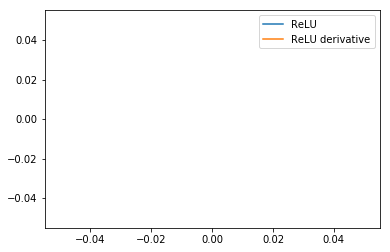### Problem 2.3¶

The softmax function

$$\sigma(\mathbf{x}) = \frac{e^{\mathbf{x}}}{\sum_{k=0}^{d-1} e^{x_k}}$$

has no parameters, so a class SoftmaxLayer that implements it can inherit dfdw from Layer.

The softmax is a function $\mathbb{R}^d\rightarrow\mathbb{R}^d$, and its Jacobian $J_{\sigma}$ with respect to $\mathbf{x}$ is therefore $d\times d$.

Show that if $\mathbf{s} = \sigma(\mathbf{x})$ then $$J_{\sigma} = \text{diag}(\mathbf{s}) - \mathbf{s}\, \mathbf{s}^T$$ where $\text{diag}(\mathbf{s})$ is a square matrix with the entries of $\mathbf{s}$ on its main diagonal.

Hint: Compute a formula for a generic entry on the diagonal and one for a generic entry off the diagonal.

### Problem 2.4¶

Show that if $c$ is any real number and $\sigma(\mathbf{x})$ is the softmax function, then $$\sigma(\mathbf{x} - c) = \sigma(\mathbf{x})\;.$$

Subtracting a scalar from a vector subtracts the scalar from each entry of the vector.

### Problem 2.5¶

The code below is a partial implementation of a softmax layer. Replace the pass command with code so that the SoftmaxLayer class works correctly.

The function f is fully implemented. The subtraction of np.max(x) from x in __softmax does not change the result (from the previous problem), and ensures that the exponents do not grow too large, thereby avoiding numerical overflow.

In :
class SoftmaxLayer(Layer):

def __softmax(x):
e = np.exp(x - np.max(x))
return e / np.sum(e)

def __init__(self, n):

def f(x):
self.x = tensorize(x)
return SoftmaxLayer.__softmax(self.x)

def dfdx():
assert self.x is not None, 'dfdx called before f'
s = SoftmaxLayer.__softmax(self.x)
pass

Layer.__init__(self, [], f, dfdx)



Similar remarks as for ReLULayer concerning the test code below.

In :
try:
ps = np.linspace(-2, 2, 101)
q = 0
softmax = SoftmaxLayer(2)
out = [(softmax.f((p, q)), softmax.dfdx()) for p in ps]

y0 = [a for a in out]
y1 = [a for a in out]
dydx00 = [a[0, 0] for a in out]
dydx01 = [a[0, 1] for a in out]

plt.figure()
plt.plot(ps, y0, label='y0')
plt.plot(ps, y1, label='y1')
plt.plot(ps, dydx00, label='dy0/dx0')
plt.plot(ps, dydx01, label='dy0/dx1')
plt.xlabel('x0')
plt.legend()
plt.show()
except:
pass


### Problem 2.6¶

The code below is a partial implementation of a Loss class that embodies the cross-entropy loss and its Jacobian. Replace the two pass commands with code so that the Loss class works correctly.

• The loss function is not part of the network, so this class is not a Layer.

• For consistency with the lecture notes, the second argument to the loss function is called p, and is the output from the network's softmax layer. The first argument is y, the true label.

• This class still conforms to the convention that method f remembers its input p in self.p, for later use by dfdx. The true label y is not remembered across calls.

• Use the natural logarithm for the cross-entropy loss.

• The value of py is clipped to self.small to prevent numerical overflow.

In :
class Loss:
def __init__(self):
self.small = 1e-8

def f(self, y, p):
self.p = tensorize(p)
py = self.p[int(y)]
if py < self.small: py = self.small
pass

def dfdx(self, y):
assert self.p is not None, 'dfdx called before f'
y = int(y)
d = np.zeros(len(self.p))
py = self.p[y]
if py < self.small: py = self.small
pass


Similar remarks as for ReLULayer concerning the test code below.

In :
try:
loss = Loss()
l, d, y = [], [], 0
xs = np.linspace(0, 1, 301)
for x in xs:
p = (x, 1-x)
l.append(loss.f(y, p))
dfdx = loss.dfdx(y)
if dfdx is not None: d.append(dfdx[y])

plt.figure()
plt.plot(xs, l, label='loss')
plt.plot(xs, d, label='loss derivative')
plt.ylim((-20, 20))
plt.legend()
plt.show()
except:
pass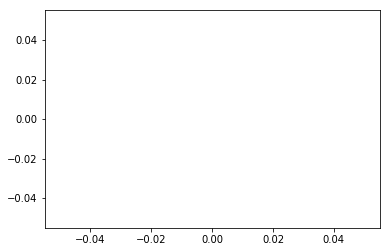## Part 3: Back-Propagation and SGD¶

This part uses the layers developed in the previous part to build a neural network. The network is limited, in that it is made of fully connected layers, ReLUs, and a softmax function. Your job is to implement back-propagation and stochastic gradient descent for this network.

### Problem 3.1¶

The code below is a partial implementation of a neural network. The only missing code is part of the backprop method that implements back-propagation. As usual, this part is replaced by a pass statement. Write code in place of this pass so that backprop computes its output by back-propagation.

• The method takes a single training data input x, its corresponding true label y, and the instance loss of class Loss.

• The method returns a pair. The first element is the value L of the loss, and the second is the gradient of the loss with respect to a vector containing all the network parameters. The entries in this vector are in the order in which they would be returned by the network's getWeights method.

• The one instruction already implemented in backprop is the forward pass through the network. This will store all layer inputs in the appropriate places.

• The lecture notes on training neural nets by back-propagation mention two Jacobians matrices in equation (5). These matrices are respectively layer.dfdw() and layer.dfdx() if layer is the current layer (layer number $k$ in the notes). Note that $\mathbf{x}^{(k)}$ is the output from that layer in the lecture notes, and the input is $\mathbf{x}^{(k-1)}$.

• Rewrite the equations for back-propagation (equations (4), (2), (3), used in this order) in terms of the code notation before attempting to write code. Do not hand in your re-writing.

• Remember that some layers do not have parameters, so there is no point in recording the corresponding Jacobians. The simplest way to tell that there are no parameters is to check that the result of calling layer.dfdw() has size zero.

• This function takes some thinking to write, but is rather simple. My replacement for the pass is eight lines of code.

In :
class Network:

def __init__(self, sizes):
self.layers = []
for i in range(len(sizes) - 1):
self.layers.append(FCLayer.ofShape(sizes[i], sizes[i+1]))
self.layers.append(ReLULayer())
self.layers.append(SoftmaxLayer(sizes[-1]))
self.p = None

def reset(self, r=None):
for layer in self.layers: layer.reset(r)
self.p = None

def getWeights(self):
return np.concatenate([layer.getWeights() for layer in self.layers])

def setWeights(self, w):
for layer in self.layers:
n = len(layer.getWeights())
layer.setWeights(w[:n])
w = w[n:]

def f(self, x):
x = tensorize(x)
for layer in self.layers: x = layer.f(x)
self.p = x
return self.p

def backprop(self, x, y, loss):
L = loss.f(y, self.f(x))
pass


Similar remarks as for ReLULayer concerning the test code below.

This code tests back-propagation for a tiny network and on a single input x. The weights are set to simple values (see the definition of w at the top), so you can also check things by hand when you debug your code.

The gradient returned by backprop is compared against the gradient computed numerically, using the Jacobian function from a previous assignment. This function would be prohibitively slow to use for larger networks.

The "Gradient gap" printed by the test code is the norm of the difference between the two results, and should be much smaller than $10^{-5}$.

In :
try:
net = Network((3, 2, 3))
L, x, y, w = Loss(), [0.1, -0.2, 0.3], 0, range(len(net.getWeights()))
net.setWeights(w)

def Lw(w):
net.setWeights(w)
return L.f(y, net.f(x))

def Jacobian(f, z, delta=1e-5):
return np.transpose([(f(z + delta * e) - f(z - delta * e)) / (2 * delta)\
for e in np.eye(len(z))])

net.setWeights(w)
backprop = net.backprop(x, y, L)
numerical = Jacobian(Lw, w)
backprop = backprop
print('Gradient gap for Network:', np.linalg.norm(backprop - numerical))
except:
pass


### Problem 3.2¶

def sgd(net, loss, T, batch_size=1, max_iter=1, learning_rate_init=1e-3,
tol=1e-6, n_iter_no_change=10):



that uses your Network.backprop function to implement stochastic gradient descent without momentum:

$$\mathbf{w}_{t+1} = \mathbf{w}_t - r \nabla\mathbf{w}_t \;.$$

where the gradient is computed on mini-batches.

Place your code in a separate notebook cell before the test code.

• net is an instance of your Network class, loss is an instance of your Loss class, and T is a training set specified as a dictionary with keywords 'x' and 'y'(you will use the provided training set T)

• The keyword parameters batch_size, max_iter, learning_rate_init, tol, and iter_no_change have the same meaning as the corresponding parameters in sklearn.neural_network.MLPClassifier. Refer to the manual page for that function to determine the meaning of these parameters, so you know how to implement their funcitonality.

• The learning rate $r$ is equal to learning_rate_init and never changes.

• The function sgd does not initialize the network parameters. Creating an instance of Network already does that.

• Your function should return a list or array of the training risk $L_T$ at the end of every epoch, which the test code will plot.

• Your function should scramble the training data randomly once, at the beginning. Do not re-scramble in each epoch. You may either scramble the data itself, or create a scrambled list or array of indices into it.

#### Programming Notes¶

The initial value of $\mathbf{w}$ can be read with the call net.getWeights(), and the updated value can be written into net with the call net.setWeights(w).

A simple way to generate an array of scrambled indices into T is as follows:

n = len(T['y'])
idx = np.argsort(np.random.random(n))



The simplest way to access the mini-batches in sequence is to use a Python generator. If you don't know what that means, just write ordinary code with a for loop.

A risk is an average loss, so remember to normalize properly.

What the MLPClassifier calls an "iteration" is an epoch, that is, a single run through all the mini-batches from the training set.

The implementation of Network is inefficient, and this is why the test code only runs 200 epochs of training. You should not expect training to achieve a small risk at the end of this small number of iterations. If you are curious, run your code longer, but do not turn in the result of that.

Since it takes a couple of minutes for the test code to run, you may want to add some progress report instructions to your code, so you see what is going on. However, this is not required.

The test code assumes that the files x and y provided with this assignment are in the same directory as your notebook.

The network created in the test code is very similar, but not identical, to the network you will work with in part 4.

## Part 4: Training a Small Network¶

In this part, you will use the simple MLPClassifier neural network made available in sklearn.neural_network. This implementation is much more efficient than the one you developed in the parts above. In addition, using pre-made code makes sure you can solve the problems below even if your earlier code does not work.

This part uses the state-of-the-art Adam optimizer to train a network. Adam is a variant of Stochastic Gradient Descent (SGD) that evaluates the step size adaptively. Convergence is typically much faster than with standard SGD, even if the Adam code is quite simple. See this ArXiV paper if you are interested in the details.

Assuming that the appropriate files are in the same directory as your notebook, the following code loads some simple training and test datasets.

The data space $X$ is two-dimensional, so we can draw the decision regions by the usual method of classifying every point on a fine grid. The true decision regions are displayed below.

In :
def readArray(filename):
with open(filename, 'r') as file:
X = np.array([[float(a) for a in line.strip().split()] for line in file])
return X

T = {'x': X, 'y': y}
S = {'x': XTest, 'y': yTest, 'shape': (50, 50)}
SGrid = {'x0': S['x'][:, 0].reshape(S['shape']),
'x1': S['x'][:, 1].reshape(S['shape']),
'y': S['y'].reshape(S['shape'])}

def showRegions(grid, title, s = None):
if s:
plt.subplot(2, 3, s)
else:
plt.figure()
plt.contourf(grid['x0'], grid['x1'], grid['y'],
cmap=plt.cm.RdBu, alpha=0.3)
plt.axis('equal')
plt.axis('off')
plt.title(title)

plt.figure(1)
showRegions(SGrid, 'True Regions')

<Figure size 432x288 with 0 Axes>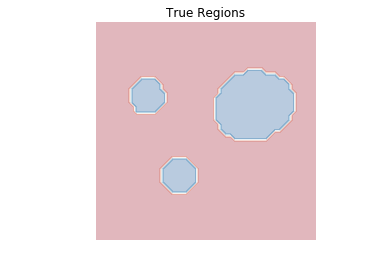### Problem 4.1¶

The code below runs exactly the same training procedure on exactly the same data six times, each time using a different random seed for initialization. The resulting decision regions are displayed. The code may take a minute or so to run on a laptop.

In :
from sklearn.neural_network import MLPClassifier

layerSizes = (16, 16, 2)
SHatGrid = SGrid
state = (2, 3, 6, 12, 13, 9)
lossCurve = []
plt.figure(figsize=(10,10))
for k in range(len(state)):
network = MLPClassifier(hidden_layer_sizes = layerSizes, tol=1e-6,
max_iter = 10000, random_state=state[k], verbose=False)
network.fit(T['x'], T['y'])
lossCurve.append(network.loss_curve_)
SHatGrid['y'] = network.predict(S['x']).reshape(S['shape'])
showRegions(SHatGrid, 'Predicted Regions ' + str(state[k]), k+1)
plt.show()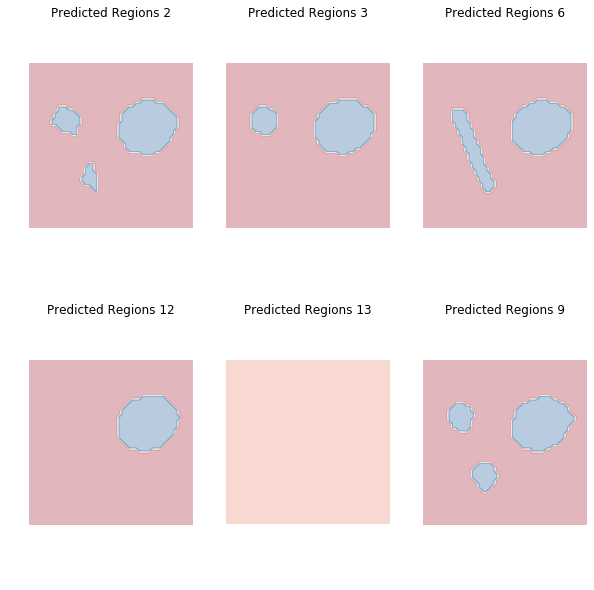Comment briefly on the importance of initialization when training a neural network. Why is this an issue in terms of advancing our empirical understanding of neural networks? Keep in mind that for larger networks training can take days or weeks, even on a cluster of GPUs.

### Problem 4.2¶

The following code displays the histories of the training risk recorded in the previous experiment.

In :
plt.figure()
for k in range(len(lossCurve)):
plt.plot(lossCurve[k], label=str(state[k]))
plt.xlabel('epoch')
plt.ylabel('training risk')
plt.legend()
plt.show()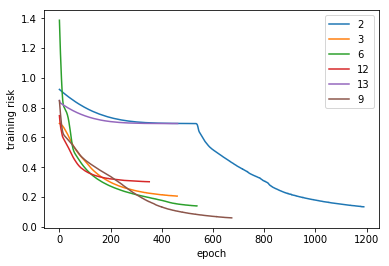In what way are these plots consistent with the decision regions displayed earlier? Comment in particular about the experiment with random_state=9.